## Quiz 30 : Aggregate Demand and Aggregate SupplyLooking for Economics Homework Help?# Quiz 30 : Aggregate Demand and Aggregate Supply

To calculate the increase in government spending we should calculate the multiplier,To calculate the desired tax cut to shift the GDP curve towards its right, we should calculate the tax multiplier.One possible combination: A \$1 billion increase in government spending can increase the GDP by \$5 billions and a \$5 billion tax cut.The central thrust of the Employment Act of 1946 specifies that the government does have a role to play in stabilizing economic conditions: prices (purchasing power), employment, and output. Council of Economic Advisors (CEA) advises and assists the president on economic maters. This council of economists advises the Federal government on policy related matters such as discretionary changes in government spending and taxes. The staff of the council includes about 20 academic economists, plus three permanent economic statisticians.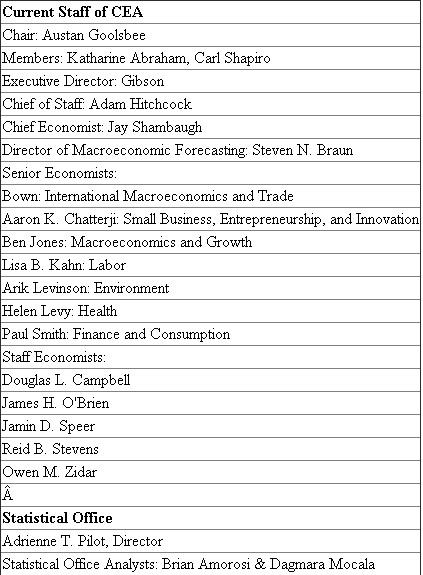First, according to figure 12.7, if aggregate demand shifted to the right by \$14 billion (suppose there is an aggregate demand curve at point a , where the real domestic output is \$502 billion), then, real domestic output will only go up by \$8 billion (from \$502 billion to \$510 billion). Thus, any aggregate demand shift should be multiplied by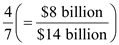to get the actual amount that the equilibrium will move. Then see that an increase of \$8 billion in real domestic output (from \$502 billion to \$510 billion) brought about an 8 percentage point increase in the price level (from 92 to 100). Thus, near the equilibrium, it appears as if a one billion dollar increase in real domestic output will change prices by one percent.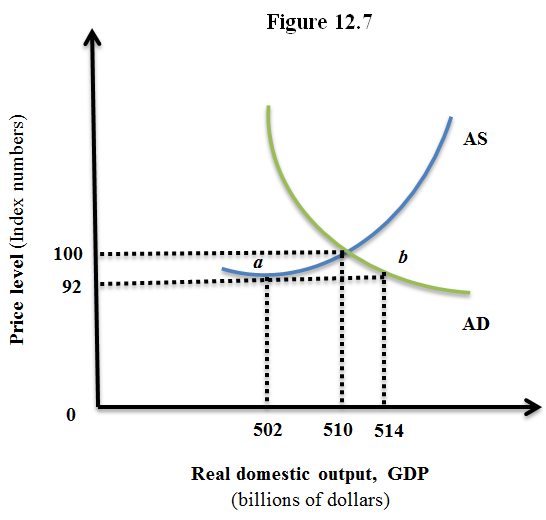If aggregate demand were to shift to the right by \$7 billion, then this would mean the equilibrium will move by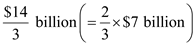Since price level will move at about a one-to-one ratio with each billion of dollar increase in real domestic output, should expect the price level to increase by 4.66 percent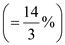. Since this is an increase in aggregate demand, it will be a demand-pull inflation. Cost-push inflation will happen if the supply side causes the inflation. However, it was given that the aggregate demand (and not the aggregate supply) increased, and thus the increase in price level is a demand-pull inflation. If the potential real GDP is \$510 billion, then the size of the positive GDP gap is \$4.66 billion, as that is the amount that the increase in aggregate demand caused the GDP to increase by (as we found earlier). If the government were to increase government spending, that would only add to the aggregate demand for the economy, since aggregate demand also includes government spending. Therefore, to offset the inflation, the government must decrease their spending. Doing so will decrease the aggregate demand and depending on the size of the decrease in spending, will offset the original increase in aggregate demand.